Next: Tidal Elongation Up: Gravitational Potential Theory Previous: Rotational Flattening

# McCullough's Formula

According to Equations (903) and (908), if the Earth is modeled as spheroid of uniform density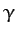then its ellipticity is given by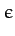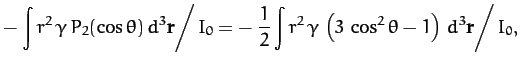(922)

where the integral is over the whole volume of the Earth, and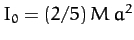would be the Earth's moment of inertia were it exactly spherical. Now, the Earth's moment of inertia about its axis of rotation is given by(923)

Here, use has been made of Equations (869)-(871). Likewise, the Earth's moment of inertia about an axis perpendicular to its axis of rotation (and passing through the Earth's center) is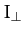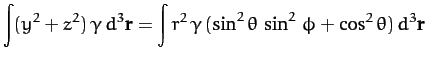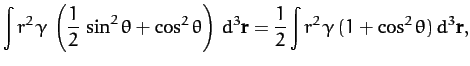(924)

since the average ofis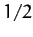for an axisymmetric mass distribution. It follows from the above three equations that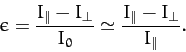(925)

This result, which is known as McCullough's formula, demonstrates that the Earth's ellipticity is directly related to the difference between its principle moments of inertia. It turns out that McCullough's formula holds for any axially symmetric mass distribution, and not just a spheroidal distribution with uniform density. Finally, McCullough's formula can be combined with Equation (909) to give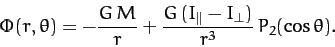(926)

This is the general expression for the gravitational potential generated outside an axially symmetric mass distribution. The first term on the right-hand side is the monopole gravitational field which would be generated if all of the mass in the distribution were concentrated at its center of mass, whereas the second term is the quadrupole field generated by any deviation from spherical symmetry in the distribution.Next: Tidal Elongation Up: Gravitational Potential Theory Previous: Rotational Flattening
Richard Fitzpatrick 2011-03-31